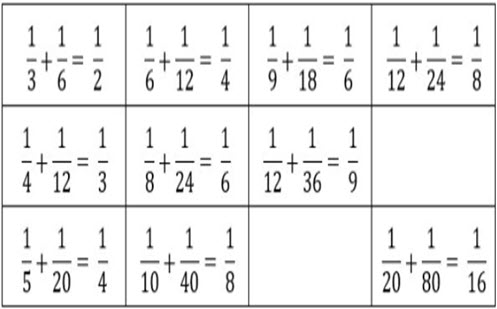Go to website

# Sum of unit fractions

This prompt invites students to look at the addition of unit fractions. It can help to reveal misconceptions students hold about fractions. The investigation can be extended to algebraic notation and proof. The resource includes classroom examples, teacher notes and guided questions.

Year level(s) Year 7
Audience Teacher
Teaching strategies and pedagogical approaches Collaborative learning, Differentiated teaching, Explicit teaching, Feedback, Mathematics investigation

## Curriculum alignment

Curriculum connections Critical and creative thinking, Numeracy
Strand and focus Number, Apply understanding, Build understanding, Algebra
Topics Fractions
AC: Mathematics (V9.0) content descriptions
AC9M7N06
Use the 4 operations with positive rational numbers including fractions, decimals and percentages to solve problems using efficient calculation strategies

Interpreting fractions (P8)
Multiplicative strategies (P10)
Number and place value (P10)
Proportional thinking (P2)